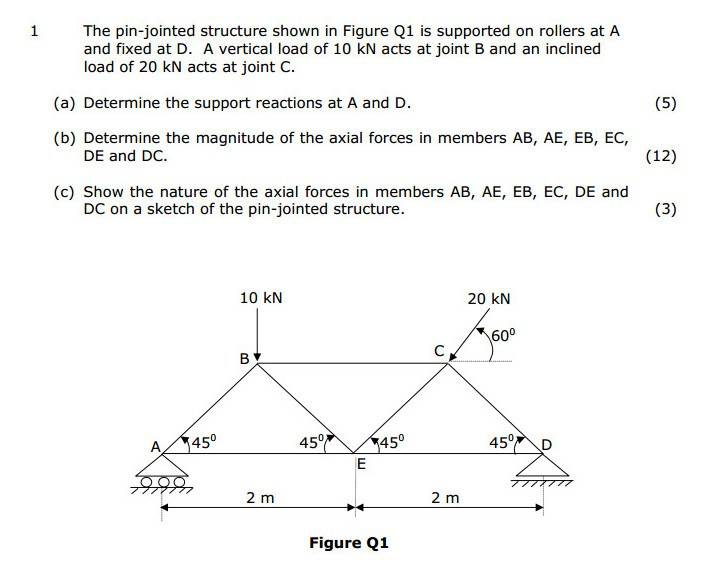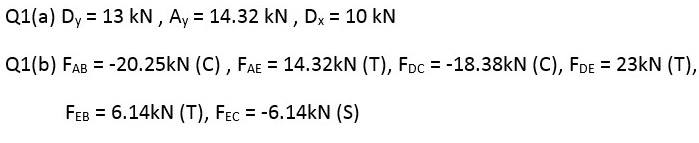# Finding Dy: Problem & Solution for Part (a) of Question

• smr101

#### smr101

Hi,

I'm looking for help with this question, part (a).

Should be noted that Cx = 10kN and Cy = 17.32kN.

Problem and solution below.I've calculated Ay and Dx succesfully but I'm having problems getting Dy. Do I need to use moments again, and if so, how?

Thanks.

yes . take moment about A. consider moment contribution at C ...both X and Y.. u need vertical distance of load that act from base.. that would be 1 . so that's it

•smr101
praveenpandiyan said:
yes . take moment about A. consider moment contribution at C ...both X and Y.. u need vertical distance of load that act from base.. that would be 1 . so that's it

OK, I've got it now, much appreciated.